Question
1. The relationship between the frequency and amplitude of the audio signal and the detected output waveform.
2. The relationship between the AM envelope and the detected waveform.
3. The causes and effects of diagonal clipping and rectifier distortion.

the relationship between frequency and amplitude in AM is independent that is there is no relationship between them.

whereas in FM frequency of the output waveform frequency increases as the amplitude of baseband signal is increased that is directly proportional relationship.

2) the relationship between AM envelop and detected waveform is directly proportional.as the amplitude of the envelope is increasing more accurate output waveform will be present at the receiver,

3) basically diagonal clipping is associated with detection of AM signal. during the detection process if the capacitor is discharging quickly then some part of the baseband signal may be lost.

we will use diodes during the detection. distortion occurred in the diode is called as rectifier distortion.bassically it is due to minority carriers in the diode.

#### Earn Coins

Coins can be redeemed for fabulous gifts.

Similar Homework Help Questions
• ### 2. An audio frequency signal 10sin2π500t) is used to amplitude modulate a carrier of 50sin(2π 100...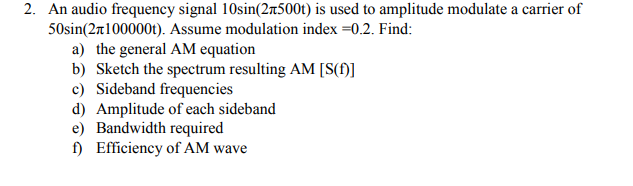Please solve whole this problem and be clear when you write by your hand. 2. An audio frequency signal 10sin2π500t) is used to amplitude modulate a carrier of 50sin(2π 100000t). Assume modulation index-02. Find: a) the general AM equation b) Sketch the spectrum resulting AM [S(] c) Sideband frequencies d) Amplitude of each sideband e) Bandwidth required f) Efficiency of AM wave 2. An audio frequency signal 10sin2π500t) is used to amplitude modulate a carrier of 50sin(2π 100000t). Assume modulation...

• ### Select the correct statement regarding the difference between the FM modulated signal waveform and the AM...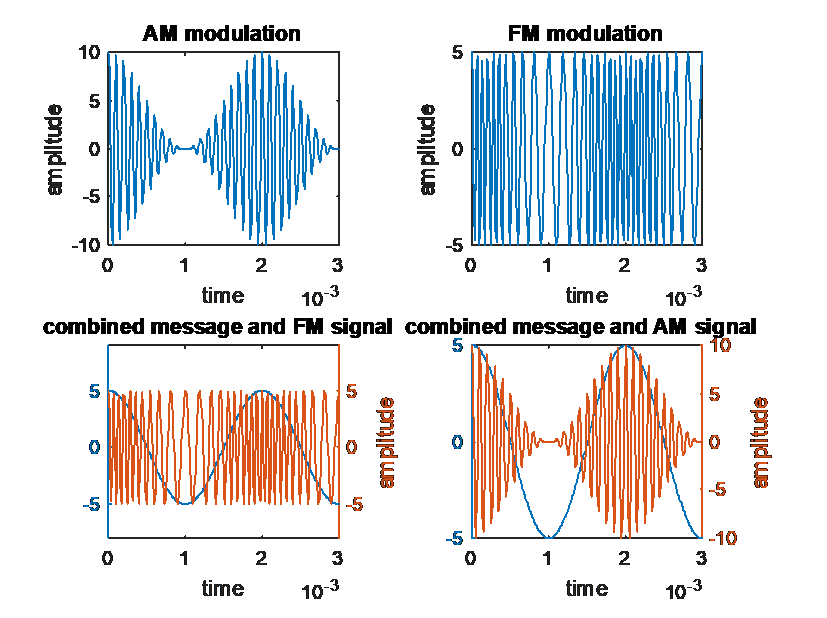Select the correct statement regarding the difference between the FM modulated signal waveform and the AM modulated signal waveform. a. The FM signal wave changes frequency according to the message wave m(t). The "peak" amplitude of the FM signal, Ac=5, remains constant. b. There is no difference between the FM and AM signal waves because they are both modulated by the same message wave, m(t) = Amcos(2πfmt) c. The AM signal wave frequency changes with the message wave m(t) while...

• ### For the AM waveform s(t) shown, the carrier signal has a peak amplitude of 0.8 V...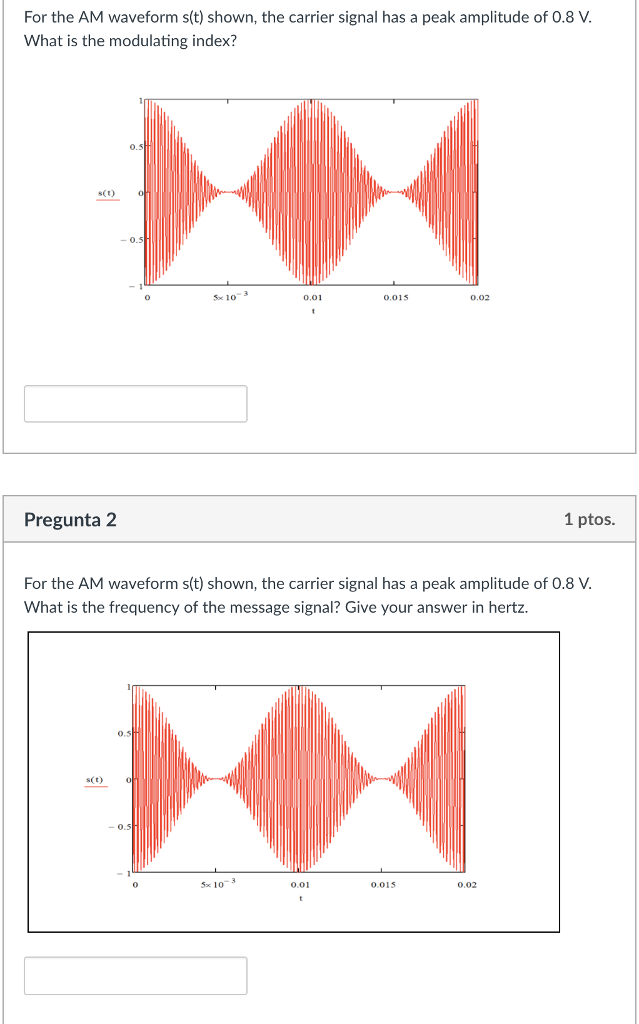For the AM waveform s(t) shown, the carrier signal has a peak amplitude of 0.8 V What is the modulating index? SCO Foc 10 0.01 0.015 0.02 Pregunta 2 1 ptos. For the AM waveform s(t) shown, the carrier signal has a peak amplitude of 0.8 V What is the frequency of the message signal? Give your answer in hertz. 5x 10-3 0.015 0.02 0.01

• ### A 1.5 MHz carrier frequency is amplitude-modulated by a 200 kHz sinewave audio signal. The bandwidth...

A 1.5 MHz carrier frequency is amplitude-modulated by a 200 kHz sinewave audio signal. The bandwidth would be A. 40 kHz. B. 405 kHz. C. 4,000 kHz. D. 400 kHz.

• ### AMPLITUDE MODULATION 1. Study in time and frequency domains of AM signals This AM signal is...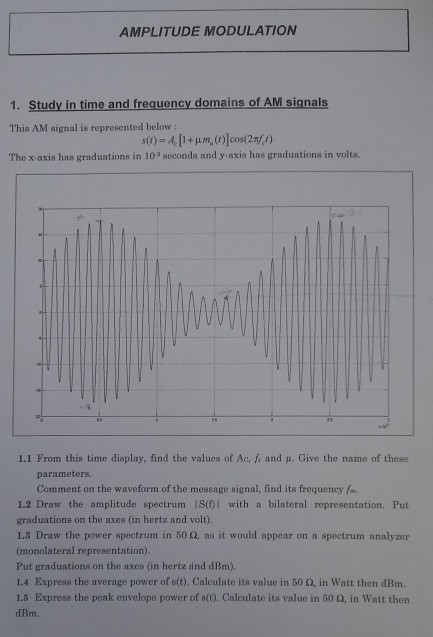AMPLITUDE MODULATION 1. Study in time and frequency domains of AM signals This AM signal is represented below The x-axis has graduations in 10 seconds and y-axis has graduations in volts. 1.1 From this time display, find the values of Ach and μ. Give the name of these parameters. Comment on the waveform of the message signal, find its frequency f 1.2 Draw the amplitude spectrum IS(DI with a bilateral representation. Put graduations on the axes (in hertz and volt)....

• ### The alternating signal with an amplitude of 0.9 V, a frequency of 1 KHz, and a phase of -22.5° is o.TV dc b.as. Try connecting this signal to a load resistance of 50 Q to test the waveform 1.E...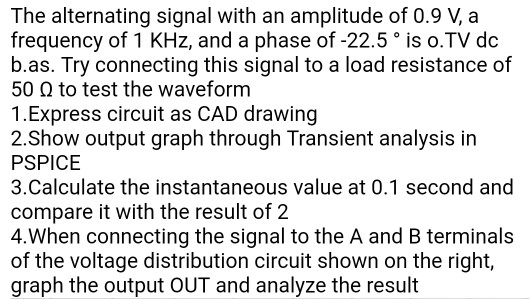The alternating signal with an amplitude of 0.9 V, a frequency of 1 KHz, and a phase of -22.5° is o.TV dc b.as. Try connecting this signal to a load resistance of 50 Q to test the waveform 1.Express circuit as CAD drawing 2.Show output graph through Transient analysis in PSPICE 3.Calculate the instantaneous value at 0.1 second and compare it with the result of 2 4.When connecting the signal to the A and B terminals of the voltage distribution...

• ### Consider the message signal m(t)=2cos(2001Tt)+3sin(4001Tt). Work out by hand the expected frequency spectrum and output waveform...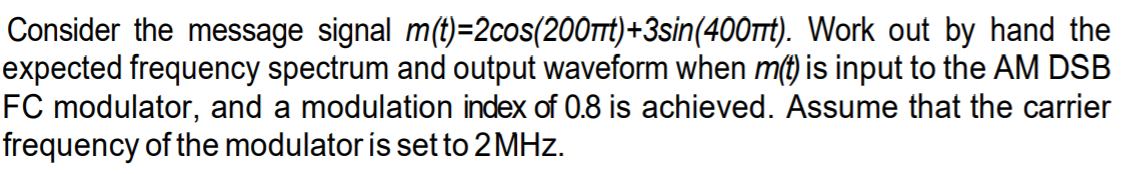Consider the message signal m(t)=2cos(2001Tt)+3sin(4001Tt). Work out by hand the expected frequency spectrum and output waveform when m(t) is input to the AM DSB FC modulator, and a modulation index of 0.8 is achieved. Assume that the carrier frequency of the modulator is set to 2 MHz.

• ### This question should be solved by MATLAB. - Generate an analog signal. (This should be an audio, any audio can be used.) - Analyze frequency spectrum of this analog signal. - Modulate it. (AM Modulati...

This question should be solved by MATLAB. - Generate an analog signal. (This should be an audio, any audio can be used.) - Analyze frequency spectrum of this analog signal. - Modulate it. (AM Modulation can be used) - Send the modulated signal over a wireless channel. (Before sending the signal, a noise should be added.) Thank you already for your help!

• ### Question 3: a.)  A signal with frequency of 9 kHz and amplitude of 200 µV is input...

Question 3: a.)  A signal with frequency of 9 kHz and amplitude of 200 µV is input to a low pass filter with cutoff frequency of 700 kHz. What are the frequency and amplitude of the output signal? b.)  A signal with frequency of 7 Hz and amplitude of 6 V is input to a low pass filter with cutoff frequency of 1 kHz. What are the frequency and amplitude of the output signal?

• ### A Pulse Amplitude Modulated (PAM) signal is generated by naturally sampling a triangular wave of amplitude 3 volt peak and frequency 1 KHz as shown in Figure 4 by a pulse train of frequency 4 kHz wit...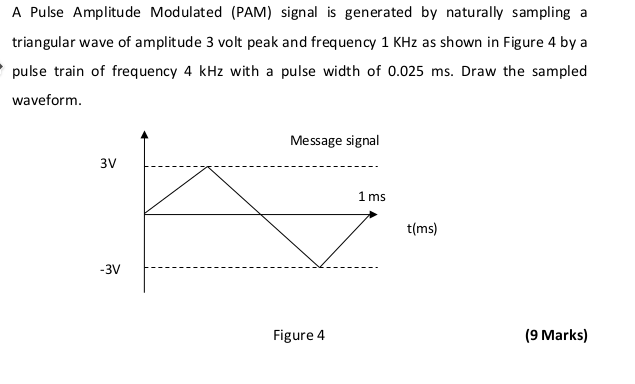A Pulse Amplitude Modulated (PAM) signal is generated by naturally sampling a triangular wave of amplitude 3 volt peak and frequency 1 KHz as shown in Figure 4 by a pulse train of frequency 4 kHz with a pulse width of 0.025 ms. Draw the sampled waveform Message signal 3V 1 ms tms 3V-- (9 Marks) Figure 4 A Pulse Amplitude Modulated (PAM) signal is generated by naturally sampling a triangular wave of amplitude 3 volt peak and frequency 1...